##### Behavioral Economics For Dummies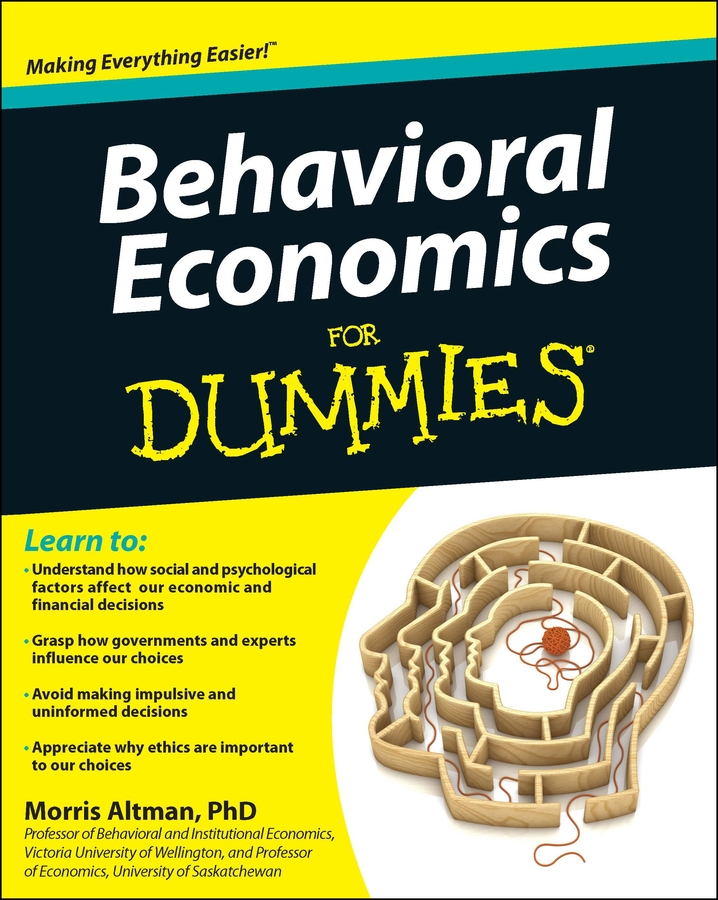There are articles that say a dollar today is worth only 25 cents. The idea of the time value of money sounds somewhat absurd, and it is. But it’s really important to recognize what happens to money over time.

A dollar today is worth — surprise — a dollar. However, a dollar today doesn’t buy as much stuff as a dollar did 30 years ago because of inflation. Given inflation, you would rather have a dollar right now, rather than a dollar ten years in the future.

Even more important than inflation is the role interest plays in the value of money. If you have a dollar today, you can use it to buy a bond and earn interest. Thus, the dollar you have today will be \$1.10 one year from now if the interest rate is 10 percent. Because of interest, you prefer receiving money now instead of in the future.

In making business decisions, it’s important that you include the time value of money — the fact that money you hold today can earn interest. Thus, if you spend money today to build a new factory, you’re giving up the opportunity to earn interest.

In the future, your factory generates profits — at least you believe it will — but you need to know whether or not those profits are large enough to offset the interest you lost by not buying a bond. This is determined by calculating the present value.

The present value of money is the value of a future stream of revenue or costs in terms of their current value. Future revenues and costs are adjusted by a discount rate that reflects the individual’s time and risk preference. Often, the discount rate is some interest rate that represents the individual’s best alternative use for money today.

The formula for calculating the present value of a future stream of net revenue — future revenues minus future costs — is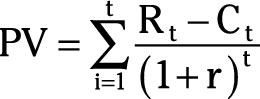where PV represents present value, Rt – Ct represents net revenue (revenue minus cost) in year t, r is the interest rate, and t is the year.

Your company accepts a contract that has an anticipated net revenue of \$100,000 at the end of each of the next three years. The interest rate is 6 percent. To determine the present value of this future stream of net revenue you take the following steps:

1. Determine the present value of year one’s net revenue.

2. Divide 100,000 by 1.06.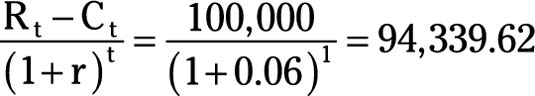3. Determine the present value of year two’s net revenue.

Divide 100,000 by (1.06)2.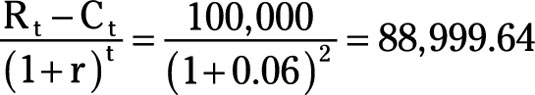4. Determine the present value of year three’s net revenue.

Divide 100,000 by (1.06)3.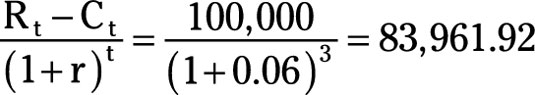5. Add the present value of net revenue for years one, two, and three.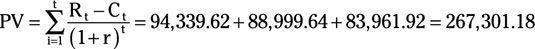Thus, the present value of \$100,000 net revenue for each of the next three years given an interest rate of 6 percent is \$267,301.18.

As an alternative to short-run profit maximization, managerial efforts can maximize the firm’s value. Focusing on maximizing the firm’s value can resolve the apparent conflict between the goal of immediate profit maximization and other goals, such as sales or growth maximization, that may increase the firm’s future profits.

A firm’s value is defined as the present value of the firm’s expected future profit, ð. Therefore,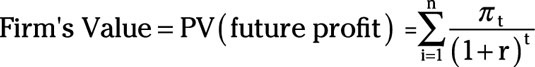where ðt represents the profit in year t, and r is the interest rate. This is simply a present value calculation that discounts profit earned in the future by the interest rate.

A number of factors influence the firm’s value. The firm’s marketing department can increase profits through various marketing strategies. Costs are frequently reduced through the firm’s engineering or production department.

Although research and development expenditures increase current costs and diminish current profits, they may result in higher future profits that more than offset those costs. Thus, maximizing the firm’s value encompasses a broad variety of strategies that you may employ as a manager.

Your ultimate goal as manager is to maximize your firm’s value. That ensures that your destiny is a good one.